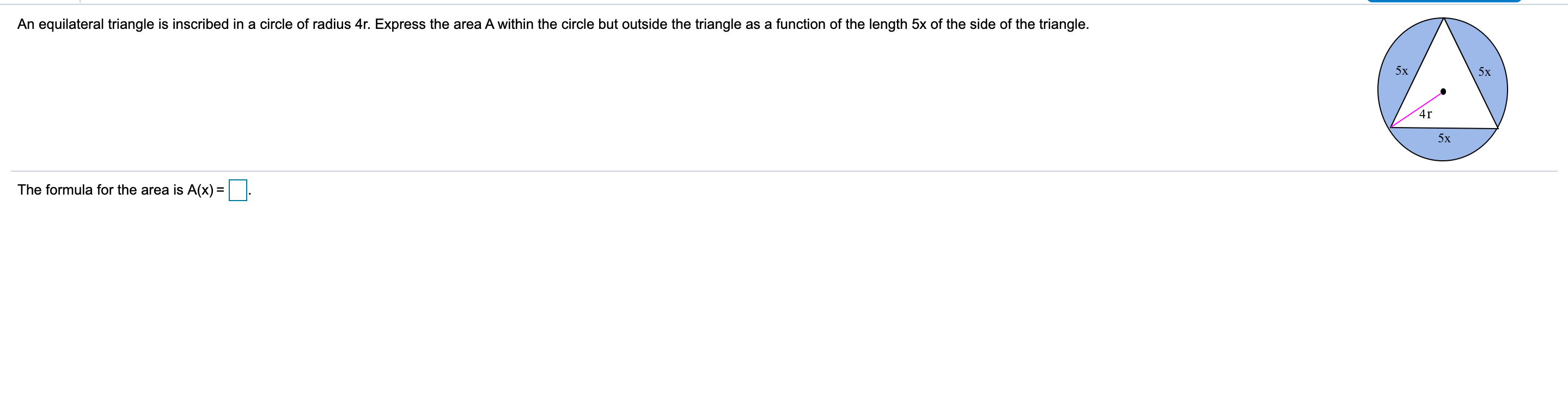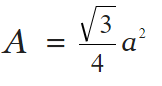# An equilateral triangle is inscribed in a circle of radius 4r. Express the area A within the circle but outside the triangle as a function of the length 5x of the side of the triangle.5x5х4r5xThe formula for the area is A(x) =||

Question
24 viewshelp_outlineImage TranscriptioncloseAn equilateral triangle is inscribed in a circle of radius 4r. Express the area A within the circle but outside the triangle as a function of the length 5x of the side of the triangle. 5x 5х 4r 5x The formula for the area is A(x) =|| fullscreen
check_circle

Step 1

We know that the area of an equilateral triangle is, where a is the length of the side.

Notice that the required area(coloured region) = Area o...

### Want to see the full answer?

See Solution

#### Want to see this answer and more?

Solutions are written by subject experts who are available 24/7. Questions are typically answered within 1 hour.*

See Solution
*Response times may vary by subject and question.
Tagged in